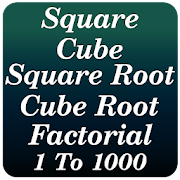# Square, Cube, Square-Root, Cube-Root & Factorial - Lumos Educational App Store4.51
Price -FREE
\$0

#### DESCRIPTION:

This app provides Square, Square Root, Cube, Cube Root & Factorial of a number from 1 to 1000.* Square (1 to 1000) * Square Root (1 to 1000) * Cube (1 to 1000) * Cube Root (1 to 1000) * Factorial (1 to 100) NOTE: Square, Cube, Square-Root, Cube-Root & Factorial is free but may contain some ads.

#### OVERVIEW:

Square, Cube, Square-Root, Cube-Root & Factorial is a free educational mobile app By Guru Balaji Developer.It helps students in grades HS practice the following standards HSN.RN.A.1.

This page not only allows students and teachers download Square, Cube, Square-Root, Cube-Root & Factorial but also find engaging Sample Questions, Videos, Pins, Worksheets, Books related to the following topics.

1. HSN.RN.A.1 : Explain how the definition of the meaning of rational exponents follows from extending the properties of integer exponents to those values, allowing for a notation for radicals in terms of rational exponents. For example, we define 51/3 to be the cube root of 5 because we want (51/3)3 = 5(1/3)3 to hold, so (51/3)3 must equal 5.

HS

#### STANDARDS:

HSN.RN.A.1

Developer: Guru Balaji Developer

Software Version: 1.1

Category: Education

### RELATED APPS